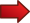# Meters per Second to Miles Per hour

Instructions: Use this step-by-step Meters per Second to Miles Per hour Calculator, to convert a speed in meters per seconds into the equivalent speed in miles per hour. Please provide the required information in the form below:Speed in meters per second (number or fraction) =

There is always confusion about the different metric systems. You have the American system, you have the imperial system, the European system. You name it.

In science, at times reluctantly, the winning convention has been the MKS system: "M" stands for meters as the measure of distance, the "K" stands for kilograms as the measure of weight, and the "S" stands for seconds as a measure of time.

That is the reason why you will sometimes want to make metric and speed conversions: Because you have a standard (MKS) and some people still use older formats. Or vice versa, you have a standard measurement, and you would like to convert it to an older format

Or you have a German machine that comes with meters per second, and you want to know the all American equivalent into miles per hour.

### Meters per Second to Miles Per hour conversion

The first question we need to answer is how to convert meters per second to miles per hour. The procedure is standard and it is always the same: Convert all the units into the desired units and simplify.

Indeed, observe that 1 mile = 1609.34 meters, and 1 hour = 60*60 = 3600 seconds

$\large \begin{array}{ccl} \displaystyle 1 \text{ } \frac{\text{mile}}{\text{hour}} & = & \displaystyle \frac{1609.34\text{ meters}}{3600 \text{ seconds}} \\ \\ & = & \displaystyle \frac{1609.34}{3600} \text{ } \frac{\text{meters}}{\text{seconds}} \\ \\ & = & \displaystyle 0.44704 \text{ } \frac{\text{meters}}{\text{seconds}} \end{array}$

Hence, solving the above equation leads to the following meters per second to miles per hour formula:

$\large \begin{array}{ccl} \displaystyle 1 \text{ } \frac{\text{meter}}{\text{second}} & = & \displaystyle\frac{1}{0.44704} \text{ } \frac{\text{mile}}{\text{hour}} \\ \\ & = & \displaystyle 2.236942 \text{ } \frac{\text{miles}}{\text{hour}} \end{array}$Then, 1 meter per second is the same as 2.236942 miles per hourIf you have a speed of $$v$$ in meters per second, then to get the equivalent speed in miles per hour you just multiply $$v$$ by 2.236942:

$2.236942 \times \text{ miles per hour}$Also, 1 mile per hour is the same as 0.44704 meters per secondIf you have a speed of $$v$$ in miles per hour, then to get the equivalent speed in meters per second you just multiply $$v$$ by 0.44704:

$0.44704 \times v \text{ meters per second}$

### Example 1

How many miles per hour are the same as 3.8 meters per second?

Solution: The speed in this case is given in meters per second, and it is 3.8 meters per second. From above, the formula is

$3.8 \text{ } \frac{\text{meter}}{\text{second}} = 3.8 \times 2.236942 \text{ } \frac{\text{miles}}{\text{hour}} = 8.50038 \text{ } \frac{\text{miles}}{\text{hour}}$

### Example 2

What is 65 miles per hour in meters per second?

Solution: Using the above formula, we get directly that 65 miles per hour is

$65 \text{ } \frac{\text{miles}}{\text{hour}} = 65 \times 0.44704 \text{ } \frac{\text{meters}}{\text{second}} = 29.05753 \text{ } \frac{\text{meters}}{\text{second}}$

### Other related conversion tools

You may be interested in conducting other types of conversions, in which case you can check out our conversion calculator tool , where you will find a large variety of conversions. In order to pass from one measure of speed to a different one, it is useful to be able to convert around different measures of distance, such as feet to miles , for example.